Analyzing High Frequency Currents and Techniques for Routing PCB Traces

This example shows how to use the RF PCB Toolbox to analyze the surface currents on the trace and focuses on the routing discipline that needs to be followed while designing the Mixed Signal PCB layout.

Build Trace

To analyze the currents on the trace, build a trace using traceLine object and visualize it.

trace = traceLine;
trace.StartPoint = [0 -20e-3];
trace.Length = [10 5*sqrt(2) 10 5*sqrt(2) 20 40 50]*1e-3;
trace.Angle  = [0 45 0 -45 0 90 180];
trace.Width  = 3e-3;
trace.Corner = "Sharp";
figure;
show(trace)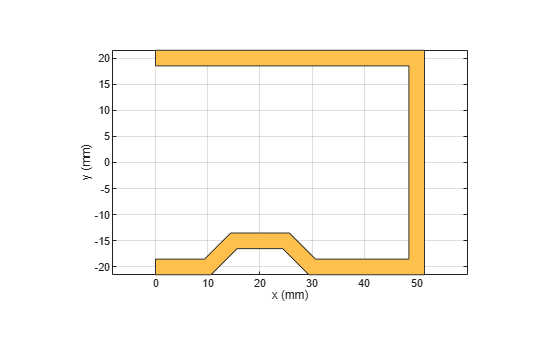Create PCB Component of Trace

Use the pcbComponent object to create the PCB stack up for the trace. Use the dielectric object function and create a teflon dielectric. Use the traceRectangular object to create the ground plane for the PCB. Assign the trace, dielectric, and the groundplane to the Layers property of the pcbComponent. Visualize the PCB stack using the show function.

pcb = pcbComponent;
d = dielectric('Teflon');
groundplane = traceRectangular('Length', 60e-3,'Width',100e-3,'Center',[60e-3/2,0]);
pcb.Layers = {trace,d,groundplane};
pcb.FeedLocations = [0e-3,-20e-3,1,3;0e-3,20e-3,1,3];
pcb.BoardShape = groundplane;
pcb.FeedDiameter = trace.Width/2;
figure;
show(pcb);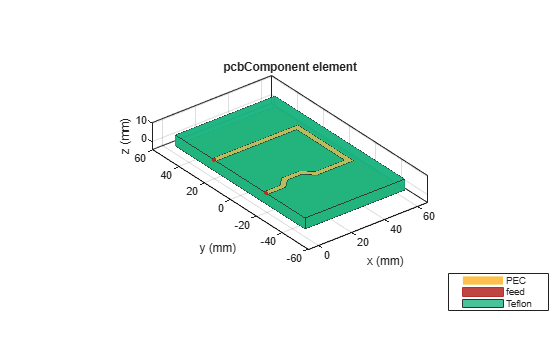Surface Currents on PCB Trace and Ground Plane

Electromagnetic simulations for signals of different frequencies are shown here to visualize the paths in which the currents flow. The forward signal currents for each case are constrained to the trace. However, the return ground currents can flow anywhere on the ground plane.

Use the current function to plot the current distribution at 100 MHz.

figure;
current(pcb,0.1e9,'scale','log');
title('Current at 100 MHz');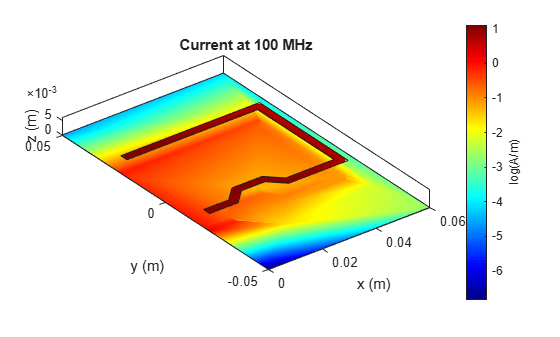The figure shows that there is a red line between the two feed locations i.e, Source and Load, which means the current on the ground plane choses the path of least resistance and returns directly from the source to the load. A small amount of the ground current flows along the signal path (light red), while even smaller amounts flow in between these two paths as indicated by the blue color of much of the plane.

As the frequency increases, the return currents on the ground plane flow exactly beneath the trace.

Use the current function to plot the current distribution at 500 MHz.

figure;
current(pcb,0.5e9,'scale','log');
title('Current at 500 MHz');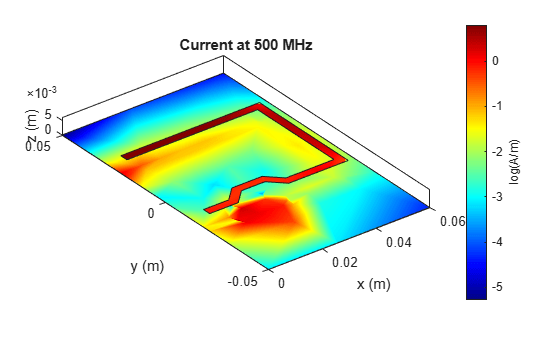This figure shows that the return current on the ground plane flows only along the trace and doesnt flow between the source and load. As the frequency increases, the return currents on the ground plane flow exactly beneath the trace and this fact can be seen in the subsequent figures.

Use the current function to plot the current distribution at 2 GHz.

figure;
current(pcb,2e9,'scale','log');
title('Current at 2 GHz');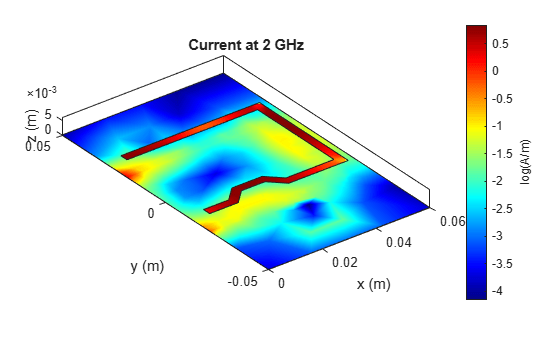This results shows that the high frequency current on the ground plane flows beneath the trace and the routing of the PCB traces on the board becomes very critical and must be followed carefully to avoid cross talk with other traces.

Routing with Gap in Ground Plane

For mixed signal PCB layout, seperate the ground plane into analog ground and digital ground to reduce the interference between these traces. But splitting the ground plane and not following the routing discipline causes severe problems in the mixed signal PCB design. To understand this, use the same trace created in the above section but with a slit in the ground plane

Use the pcbComponent object to build the PCB Stack of the trace. Use traceRectangular to build two rectangular traces and subtract these traces from the ground plane to create the slit in the ground plane and visualise it.

pcb = pcbComponent;
d = dielectric('Teflon');
groundplane1 = traceRectangular('Length', 60e-3,'Width',100e-3,'Center',[60e-3/2,0]);
slit1 = traceRectangular('Length', 1e-3,'Width',48e-3,'Center',[20e-3,27e-3]);
slit2 = traceRectangular('Length', 1e-3,'Width',50e-3,'Center',[20e-3,-27e-3]);
groundplane = groundplane1-slit1-slit2;
pcb.Layers  = {trace,d,groundplane};
pcb.FeedLocations = [0e-3,-20e-3,1,3;0e-3,20e-3,1,3];
pcb.BoardShape = groundplane1;
pcb.FeedDiameter = trace.Width/2;
figure;
show(pcb);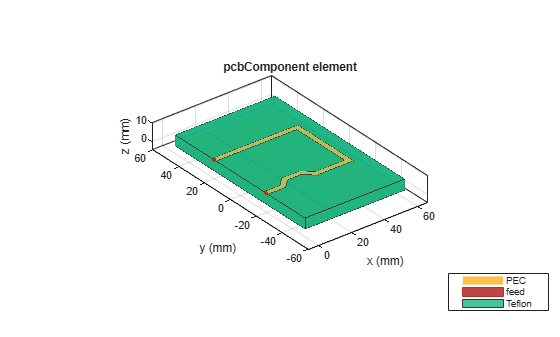The slit in the ground plane is such that the trace above crosses the slit perpendicularly as shown in the figure.

Use the current function to plot the current distribution at 5 GHz. As there is a slit in the ground plane the currents cannot return directly beneath the trace and takes a longer route to return through the small joint where both the ground planes are attached.

figure;
current(pcb,5e9,'scale','log');
title('Current at 5 GHz with Slit Ground Plane');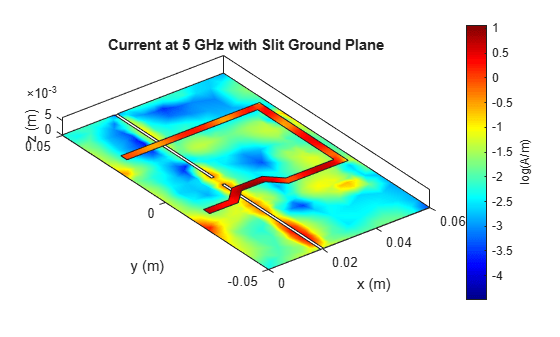The result shows that the return currents take a longer route and might interfere with the components placed in that area and introduce noise at that component. So even if the ground is seperated as an analog and digital ground, the routing of the traces is very important to design a PCB with less crosstalk and noise.

References

1. Mark Fortunato, Successful PCB Grounding with Mixed-Signal Chips - Follow the Path of Least Impedance

2. Henry W. Ott, Partitioning and Layout of a Mixed-Signal PCB.

RF PCB Toolbox Documentation